BENDING MOMENT AND SHEARING FORCE DIAGRAMS

# 1 BENDING MOMENT AND SHEARING FORCE DIAGRAMS

## 1.1 Problem definition

Verify the uprising sectional results when a constant line load stresses a bar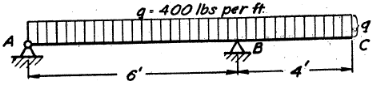Figure 1: Model by Timoshenko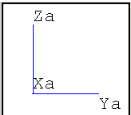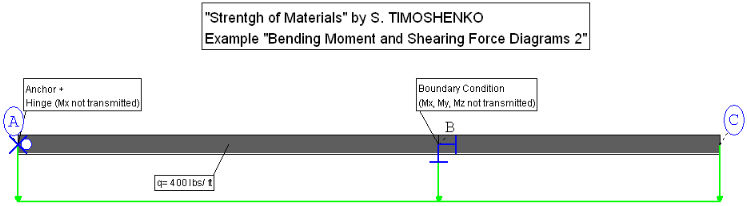Figure 2: Rohr2 Model

## 1.2 References (Timoshenko)

S. Timoshenko, Strength of Material, Part I, Elementary Theory and Problems, 3rd Edition, D. Van Nostrand Co., Inc., New York, NY, 1956, Chapter 3.22, pg. 86.

At a system with a negative line load and two boundary conditions are following parameters given:

• Constant line load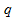• Length stretch AB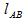• Length stretch BC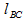• Total length (stretch AC)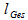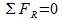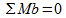Where:

 Variable Description Unit Used Value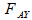Sectional force AY lbf 670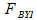Sectional force BY1 lbf -1730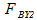Sectional force BY2 lbf 1600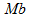Bending Moment ft lbs -3180Constant line load lbs/ ft 400LengthAB ft 6LengthBC ft 4Total length ft 10

Table 1: Overview of the used variables

## 1.3 Model description (ROHR2)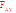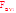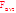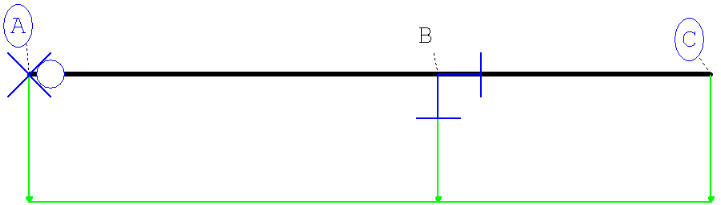Figure 3: Overview of the force vectors

This system was built of two unequal long beams. The first (stretch AB) is six feet and the second one (stretch BC) is four feet long. The profile which is used, is equal to the previous model. The ten feet long beam is supported at the point A with an anchor and at point B with an axial stop (-Z direction is also blocked). The anchor does not block the bending moment (Mx). At the whole length, the structural section is submitted to a constant line load (400 lbs/ inchÂ²). Like in the last few examples an occasional load case is creaded, without gravity. The third point C is overhanging right next to point B and was defined without any support.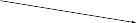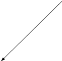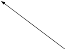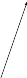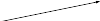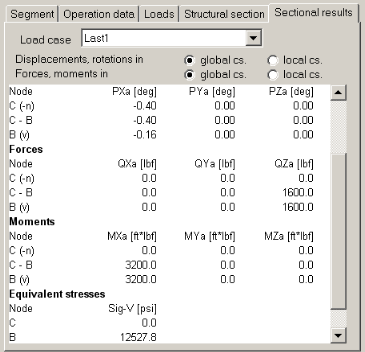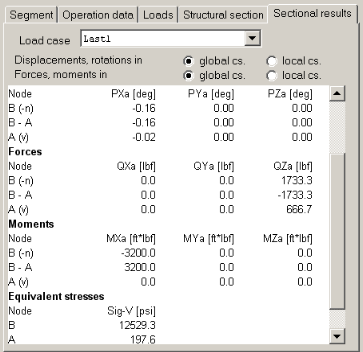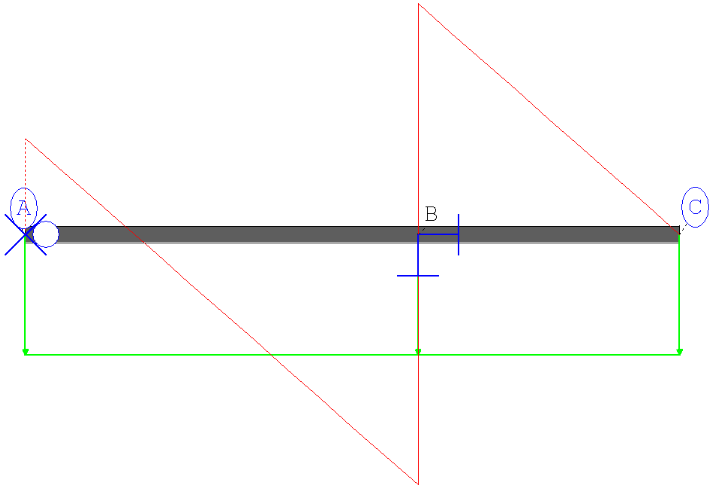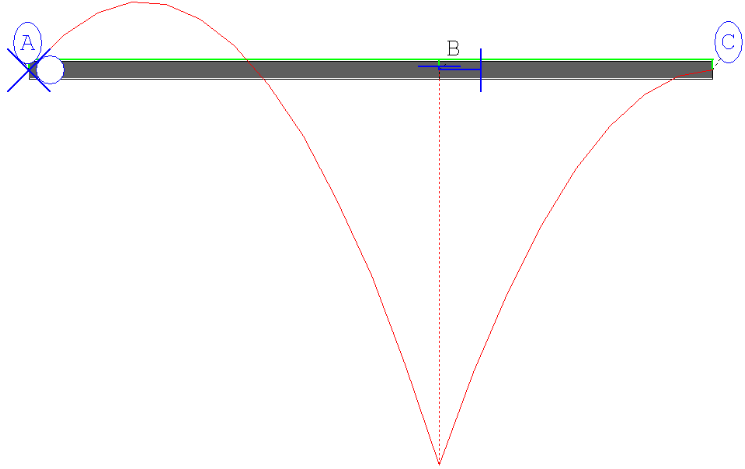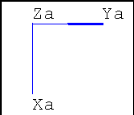Figure 4: FxY, Mb at every points of the system

## 1.4 Result comparisons

 Value Length [ft] Reference (Timoshenko) [lbf; ft*lbs] Rohr2 [lbf; ft*lbs] Difference [%]10,0 670,00 666,7 <0,52-1730,00 -1733,3 <0,191600,00 1600,0 <0,01-3180,00 -3200,0 <0,63

Table 2: Comparison of the sectional results

## 1.5 Conclusion

The results are really close to the reference. The results would be exactly in the limit of the given digits, if Mr. S. Timoshenko didn't round up the loads. It can be remarked, that the dimensions, lengths and materials are free selectable as they don't influence the results checked.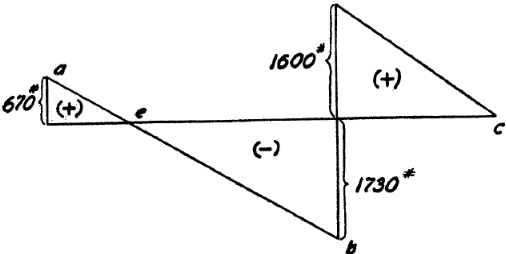Figure 5: force curve by Timoshenko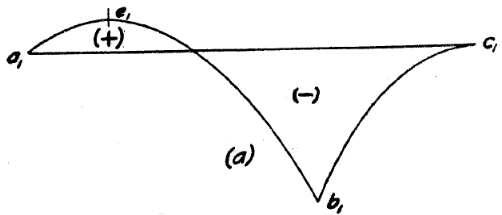Figure 6: moment curve by Timoshenko

## 1.6 Files

R008_inch.r2w

R008_mm.r2w

R2_stresses_8

SIGMA Ingenieurgesellschaft mbH www.rohr2.com